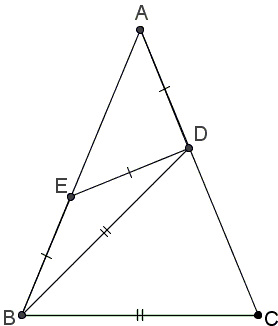A Decomposition of Isosceles Triangles into 4 Isosecles Triangles

The applet below has been inspired by the Consecutive Isosceles Decomposition of isosceles triangles and a question posted by David Radcliffe on twitter.com.

David asked to find the apex angle in the following configuration:where ABC is an isosceles triangle. It is not difficult (by "angle chasing") to see that, in David's problem the apex angle is 45°

However, as you may discover playing with the applet, that David's problem admits an extension.

 What if applet does not run?

Discussion

In an isosceles triangle ABC, with AB = AC, let D on AB be such that CD = BC; E on AC be such that CE = DE; F on AB satisfy DE = EF; and, finally, AF = EF. Find the apex angle at A.

 What if applet does not run?

The curious thing about this construction is that - apart from 45° - the construction goes through for 0 < ∠A < 60°. So that any such angle answers the question.

For angles below 45° the construction provides a decomposition of an isosceles triangle into 4 isosceles triangles. For 45° the decomposition consists of 3 isosceles triangles. For larger angles up to 60° The construction works but it's no longer a decomposition as some lines intersect.

It may be disheartening to be unable to point out a specific angle as a solution to a problem. We'll get a unique solution adding constraints to the configuration. For example, it is easily seen that there are two obtuse (CDE and AEF) and two acute (BCD and DEF) triangles.

For what apex angle the triangles in one of the pairs are similar?

Let's denote the apex angle in ΔABC α. Then

in ΔAEF, ∠EAF = ∠FEA = α, ∠AFE = 180° - 2α;
in ΔEFD, ∠DFE = ∠EFD = 2α, ∠DEF = 180° - 4α;
in ΔCDE, ∠CED = 3α, ∠CDE = ∠DCE = 90° - 3α/2;
in ΔBCD, ∠CBD = ∠CDB = 90° - α/2, ∠BCD = α.

For triangles CDE and AEF to be similar one needs

180° - 2α = 3α,

making α = 36°. For triangles BCD and DEF to be similar one needs

180° - 4α = α,

again making α = 36°. Either both pairs of triangles consist of similar triangles or both are not. In any event, this happens when ABC is a Golden Triangle.